# 3.2 The 4th degree conoid

Revise the properties of the 4th degree conoid (EXAMPLE 2 - in Croatian).

#### 3.2.1 Normal curvatures, asymptotic and principal directions

The singular points of that conoid lie on two straight lines - one of them is at infinity. All other points of the conoid are regular. The tangent plane at a regular point T cuts the conoid into the ruling and 3rd order curve. The normal line n through T is perpendicular to the tangent plane t T. The pencil of lines (T) in the plane t T contains the tangent lines to all curves on the conoid which pass through a point T.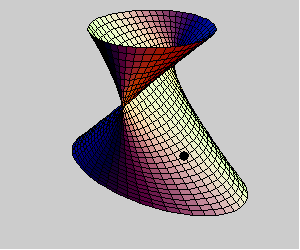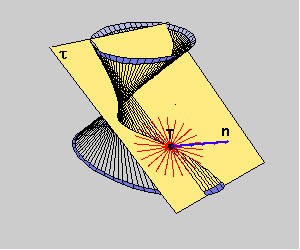We observe the pencil of planes [n] and the curvatures of the normal sections in those planes. The normal curvature of the conoid at a point T is a function which has maximum and minimum at the segment [0,p]. The angles for those extremal values correspond to the principal directions, and the angels where normal curvature is zero correspond to the asymptotic directions.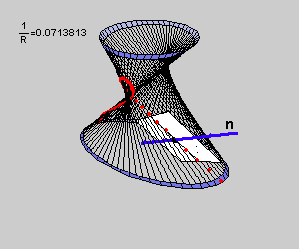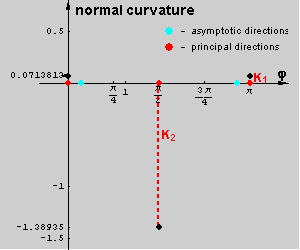The asymptotic directions at a regular point T are defined by ruling and the tangent line t of the intersection curve of a tangent plane and the conoid.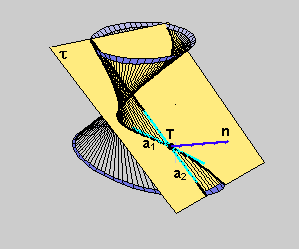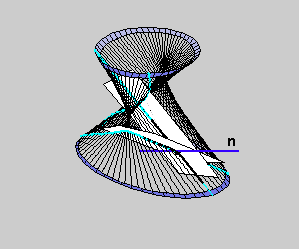The principal directions at a regular point T lying on the conoid bisect the angle between the asymptotic direction.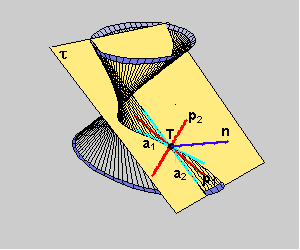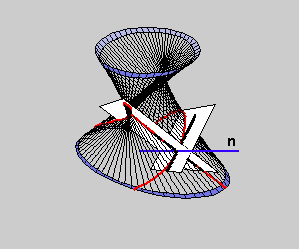The following pictures show the normal sections through the principal and asymptotic directions and the graph of the normal curvature of the conoid at its regular point T.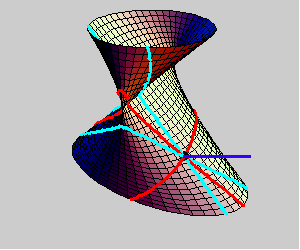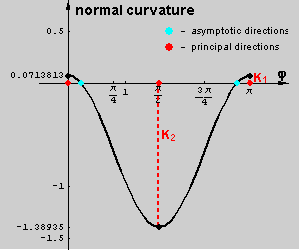#### 3.2.2 Mathematica visualisations of Gaussian and mean curvatures

For the following visualizations we used the periodical Mathematica color function Hue (period 1). It is defined in the following way: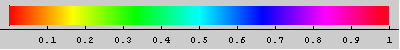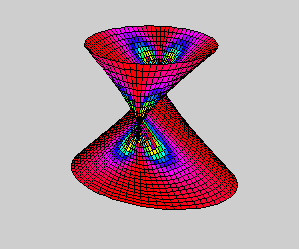the conoid coloured by Hue[G].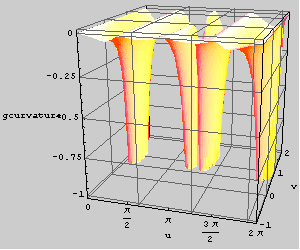The graph of the Gaussian curvature of the conoid.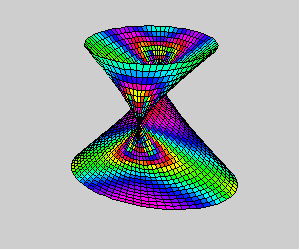the conoid coloured by Hue[H].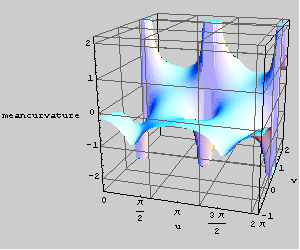The graph of the mean curvature of the conoid.

If you are inerested in Mathematica notebook containing inputs for drawing pictures and animations used in this file download slike_konoid.nb.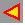back